Question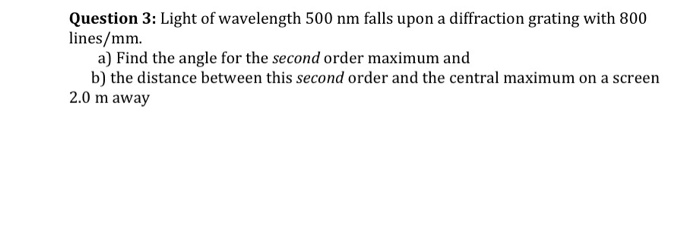a)dsin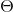=m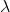Distance between 2lines in the grating,d=1/(8×10^5)

d=1.25×10^-6m , wavelength=500nm=5×10^-7m

Sin=m/d

Sin=2×5×10^-7/1.25×10^-6=0.8

Or angle=sin-1(0.8)=53.13

b)D=xtanD=2×1.333=2.666m

#### Earn Coins

Coins can be redeemed for fabulous gifts.

Similar Homework Help Questions
• ### Light of wavelength 500 nm falls on a 0.37 mm wide slit and forms a diffraction pattern on a...

Light of wavelength 500 nm falls on a 0.37 mm wide slit and forms a diffraction pattern on a screen 2.0 m away.(a) Find the position of the first dark band on each side of the central maximum.mm(b) Find the width of the central maximum.mm

• ### A 500 lines per mm diffraction grating is illuminated by light of wavelength 560 nm ....

A 500 lines per mm diffraction grating is illuminated by light of wavelength 560 nm . Part A What is the maximum diffraction order seen Part B: What is the angle of each diffraction order starting from zero diffraction order to the maximum visible diffraction order? Enter your answers in degrees in ascending order separated by commas.

• ### 2. Light of wavelength 545 nm passes through a diffraction grating with 1.35 x103 slits/cm The...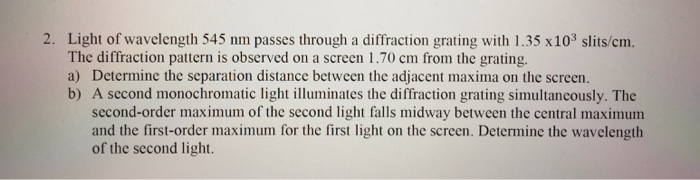2. Light of wavelength 545 nm passes through a diffraction grating with 1.35 x103 slits/cm The diffraction pattern is observed on a screen 1.70 cm from the grating. a) Determine the separation distance between the adjacent maxima on the screen. b) A second monochromatic light illuminates the diffraction grating simultancously. The second-order maximum of the second light falls midway between the central maximum and the first-order maximum for the first light on the screen. Determine the wavelength of the second...

• ### A 500 lines per mm diffraction grating is illuminated by light of wavelength 640 nm .

A 500 lines per mm diffraction grating is illuminated by light of wavelength 640 nm . For the steps and strategies involved in solving a similar problem, you may view a Video Tutor Solution.Part AWhat is the maximum diffraction order seen? Express your answer as an integer. Part B What is the angle of each diffraction order starting from zero diffraction order to the maximum visible diffraction order?

• ### Monochromatic light shines on a diffraction grating with 8,600 lines uniformly distributed over 1.8 cm. The...

Monochromatic light shines on a diffraction grating with 8,600 lines uniformly distributed over 1.8 cm. The grating is illuminated using a laser of 630 nm wavelength. A diffraction pattern is formed on a screen located at 1.7 m away from the grating. (a) What is the angle of the first-order maximum of the 630-nm light incident upon the grating? (b) What is the separation on the screen between the first and the second order maxima? (c) What is the highest...

• ### A diffraction grating with 610 lines per mm is illuminated with light of wavelength 520 nm...

A diffraction grating with 610 lines per mm is illuminated with light of wavelength 520 nm . A very wide viewing screen is 2.0 m behind the grating. Part A What is the distance between the two m=1 fringes? Express your answer in meters. ΔyΔ y = nothing m Request Answer Part B How many bright fringes can be seen on the screen? Express your answer as an integer.

• ### A 500 lines per mm diffraction grating is illuminated by light of wavelength 510...continues

A 500 lines per mm diffraction grating is illuminated by light of wavelength 510 nm. What is the angle of each diffraction order?

• ### Light of wavelength 546 nm from a mercury arc falls on a diffraction grating rul...continues

Light of wavelength 546 nm from a mercuryarc falls on a diffraction grating ruled with20400 lines/in.What is the angular separation between thefirst-order images on either side of the centralmaximum? (Caution: do not use small angleapproximation here.)Answer in units of

• ### If a diffraction grating produces a third-order bright spot for red light of wavelength 700 nm, at 65° from the central maximum at what angle will the second order bright spot be for violet light...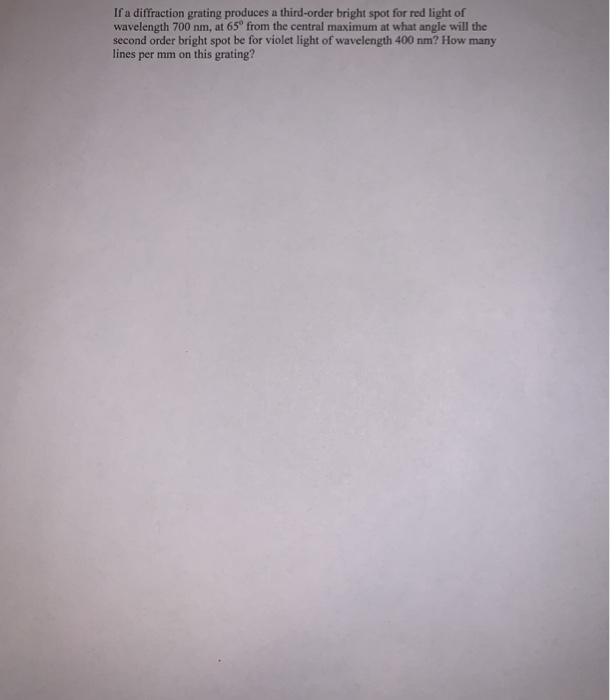If a diffraction grating produces a third-order bright spot for red light of wavelength 700 nm, at 65° from the central maximum at what angle will the second order bright spot be for violet light of wavelength 400 nm? How many lines per mm on this grating? If a diffraction grating produces a third-order bright spot for red light of wavelength 700 nm, at 65° from the central maximum at what angle will the second order bright spot be for...

• ### Light of wavelength 450 nm falls on a 0.47 mm wide slit and forms a diffraction...

Light of wavelength 450 nm falls on a 0.47 mm wide slit and forms a diffraction pattern on a screen 1.7 m away. (a) Find the position of the first dark band on each side of the central maximum. mm (b) Find the width of the central maximum. mm